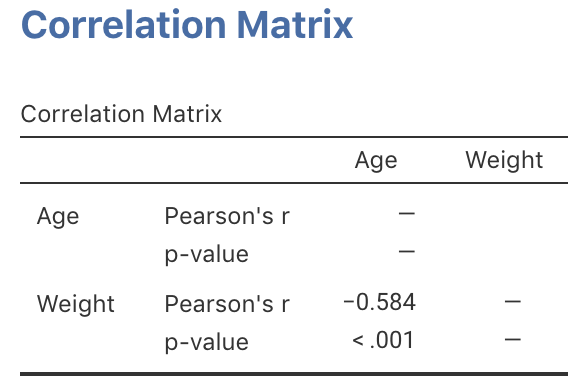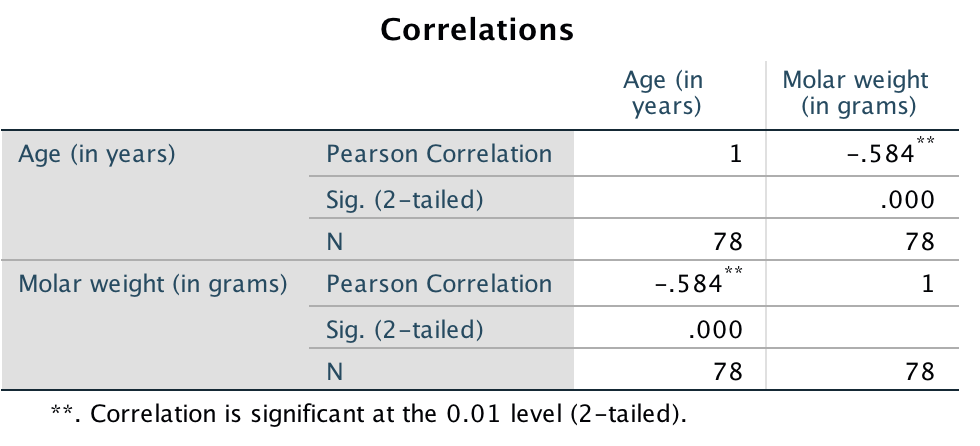## 34.2 Using software

Software is used to compute the value of $$r$$. For the red deer data (Fig. 33.2), the relationship is approximately linear, and the jamovi output (Fig. 34.5) and SPSS output (Fig. 34.6) show that $$r = -0.584$$:

• Direction: The sign of $$r$$ indicates the direction. Here we see a negative relationship: Higher ages are associated with lighter molars (in general), which makes sense.
• Variation: The value of $$r$$ indicates the strength of the relationship. Here, perhaps we could describe the variation as moderate.FIGURE 34.5: jamovi correlation output for the red deer dataFIGURE 34.6: SPSS correlation output for the red deer data